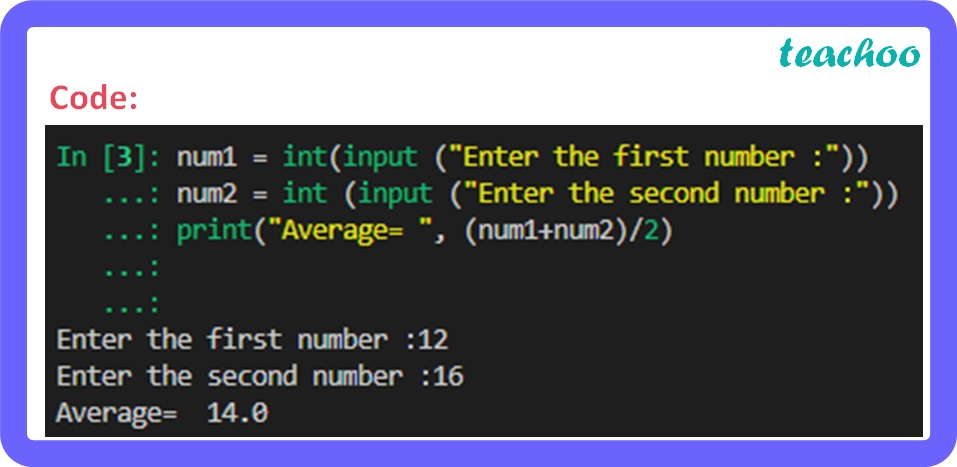Short Answer Type Questions (2 Marks each)

Computer Science - Class 11
Chapter 5 Class 11 - Getting started with python

## Write a program that computes the average of two numbers that the user enters.

Code:

``` num1 = int(input ( "Enter the first number :" ))  ```

``` num2 = int (input ( "Enter the second number :" ))  ```

``` print( "Average= " , (num1+num2)/ 2 ) ```Learn in your speed, with individual attention - Teachoo Maths 1-on-1 Class